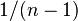# Multiple-choice question

(diff) ← Older revision | Latest revision (diff) | Newer revision → (diff)

## Definition

A multiple-choice question' (MCQ) is a question where multiple choices (also called options) are provided, and the respondent is expected to select one or more of the options based on the question.

Multiple-choice questions could be:

• questions where respondents are expected to pick a single response
• questions where respondents are expected to pick one or more options in response

Further, they could involve:

• a pre-specified correct answer option (or option set) against which the respondent's option is compared
• a survey multiple-choice question, that is intended to get information about the respondent's preference or individual-specific information. In this case, there is no right answer.

This page is not about survey MCQs. Rather, it is about questions where there is a single specific correct answer. For clarity, we will restrict attention to the case where respondents are expected to pick a single response. Cases where respondents need to pick sets of options can be reduced to this case through a mathematical equivalence.

## Related concepts

### Controlling for random guesses: the basic model

Here, we assume no scoring penalty for random guesses, and we assume that respondents attempt every question.

The simplest model for controlling for random guesses is that for every question, every respondent falls into one of two categories:

• The respondent knows the correct answer
• The respondent makes a guess that has a$1/n$ probability of being correct where$n$ is the number of options

If we follow this model, then:

Expected number of answers the respondent does not know =$n/(n - 1)$ * (Apparent number of wrong answers)

Or equivalently:

Expected number of answers the respondent knows = Number of answers the respondent gets right -$1/(n - 1)$ * (Number of answers the respondent get wrong)

Similar formulas apply to the study of a single question across multiple respondents.

The basic model is unrealistic for a number of reasons, namely that people often have specific misconceptions or make specific careless errors that lead them to select wrong answers. On the other hand, some might guess the right answer with a higher probability than mere chance through a process of elimination, without actually knowing the answer. The design of the options is extremely relevant to inferring the respondent's knowledge from the responses.